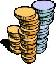Financial Terms Balloon maturity

# Definition of Balloon maturity## Balloon maturity

Any large principal payment due at maturity for a bond or loan with or without a a sinking
fund requirement.

# Related Terms:

## Sinking fund requirement

A condition included in some corporate bond indentures that requires the issuer to
retire a specified portion of debt each year. Any principal due at maturity is called the balloon maturity.

## Average maturity

The average time to maturity of securities held by a mutual fund. Changes in interest rates
have greater impact on funds with longer average life.

## Current maturity

Current time to maturity on an outstanding debt instrument.
Current / noncurrent method
Under this currency translation method, all of a foreign subsidiary's current
assets and liabilities are translated into home currency at the current exchange rate while noncurrent assets
and liabilities are translated at the historical exchange rate, that is, the rate in effect at the time the asset was
acquired or the liability incurred.

## Held-to-Maturity Security

A debt security for which the investing entity has both the positive
intent and the ability to hold until maturity.

## Maturity

For a bond, the date on which the principal is required to be repaid. In an interest rate swap, the
date that the swap stops accruing interest.

## Maturity

The date or the number of days until a security is due to be paid or
a loan is to be repaid

## Maturity

Time at which a bond can be redeemed for its face value.## Maturity

The time when a policy or annuity reaches the end of its span.

## Maturity date

The date when the issuer returns the final face value of a bond

## Maturity Date

Date on which a debt is due for payment.

## Maturity factoring

Factoring arrangement that provides collection and insurance of accounts receivable.

## Maturity phase

A phase of company development in which earnings continue to grow at the rate of the
general economy. Related: Three-phase DDM.

Extra average return from investing in longversus short-term Treasury securities.

The spread between any two maturity sectors of the bond market.

## Maturity value

Related: par value.

## Original maturity

maturity at issue. For example, a five year note has an original maturity of 5 years; one
year later it has a maturity of 4 years.

## Projected maturity date

With CMOs, final payment at the end of the estimated cash flow window.

## Remaining maturity

The length of time remaining until a bond's maturity.

## Return-to-maturity expectations

A variant of pure expectations theory which suggests that the return that an
investor will realize by rolling over short-term bonds to some investment horizon will be the same as holding
a zero-coupon bond with a maturity that is the same as that investment horizon.

## Stated maturity

For the CMO tranche, the date the last payment would occur at zero CPR.

## Term to maturity

The time remaining on a bond's life, or the date on which the debt will cease to exist and
the borrower will have completely paid off the amount borrowed. See: maturity.

## Term to Maturity

Period of time from the present to the redemption date of a bond.

## Time to maturity

The time remaining until a financial contract expires. Also called time until expiration.

## Weighted average maturity

The WAM of a MBS is the weighted average of the remaining terms to maturity
of the mortgages underlying the collateral pool at the date of issue, using as the weighting factor the balance
of each of the mortgages as of the issue date.

## Weighted average remaining maturity

The average remaining term of the mortgages underlying a MBS.

## Yield to maturity

The percentage rate of return paid on a bond, note or other fixed income security if you
buy and hold it to its maturity date. The calculation for YTM is based on the coupon rate, length of time to
maturity and market price. It assumes that coupon interest paid over the life of the bond will be reinvested at
the same rate.

## Yield to Maturity

The measure of the average rate of return that will be earned on a
debt security held until it matures

## Yield to maturity

A measure of the average rate of return that will be earned
on a bond if held to maturity.

## yield to maturity

Interest rate for which the present value of the bond’s payments equals the price.